# Two months

Mars has two months, Phobos and Deimos. Phobos orbits around Mars in 7 hours 39 minutes and Deimos in 30 h 14 min. How long will repeat the relative position of the three celestial bodies?

Result

t = 13877:06#### Solution:Leave us a comment of example and its solution (i.e. if it is still somewhat unclear...):

Showing 0 comments:Be the first to comment!#### To solve this example are needed these knowledge from mathematics:

Do you want to calculate least common multiple two or more numbers?

## Next similar examples:

1. Trams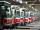Two trams started at the same time from the same place. One tram journey takes 30 minutes and the second 45 minutes to its final stop. How long will trams meet again?
2. Theorem proveWe want to prove the sentense: If the natural number n is divisible by six, then n is divisible by three. From what assumption we started?
3. Nuts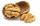How many we must have at least nuts if we can equally divide it to 10 children, 11 children or 19 children and any nut left?
4. Numbers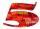Write smallest three-digit number, which in division 5 and 7 gives the rest 2.
5. Seamstress 2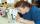Seamstress bought two kinds of textile in whole meters. One at 50 SKK and the second 70 SKK per meter. How many meter bought from this two textiles when paid totally 1540 SKK?
6. Plum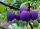On the platter are plum. How many were there if its have to be able to share equally among 8,10 and 12 children?
7. FamilyFamily has 4 children. Ondra is 3 years older than Matthew and Karlos 5 years older than the youngest Jane. We know that they are together 30 years and 3 years ago they were together 19 years. Determine how old the children are.
8. Lee isLee is 8 years more than twice Park's age, 4 years ago, Lee was three times as old. How old was Lee 4 years ago?
9. Ages 2A man's age is 4 times his son's age. After 5 years he will be just twice his son's age, find their ages.
10. One halfOne half of ? is: ?
11. Nineteenth memberFind the nineteenth member of the arithmetic sequence: a1=33 d=5 find a19
12. TreesA certain species of tree grows an average of 0.5 cm per week. Write an equation for the sequence that represents the weekly height of this tree in centimeters if the measurements begin when the tree is 200 centimeters tall.
13. Two equationsSolve equations (use adding and subtracting of linear equations): -4x+11y=5 6x-11y=-5
14. Factory and divisionsThe factory consists of three auxiliary divisions total 2,406 employees. The second division has 76 employees less than 1st division and 3rd division has 212 employees more than the 2nd. How many employees has each division?
15. 6 termsFind the first six terms of the sequence. a1 = 7, an = an-1 + 6
16. Linear systemSolve a set of two equations of two unknowns: 1.5x+1.2y=0.6 0.8x-0.2y=2
17. 15 teachers15 teachers teach for a combined amount of 128 days over a period of 64 days. What is this expressed as a percentage?